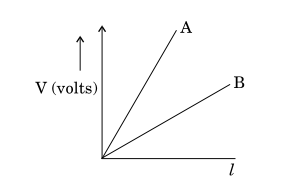# NEET Physics Current Electricity Questions Solved

(a) (i) State the principle on which a potentiometer works. How can a given potentiometer be made more sensitive?

(ii) In the graph shown below for two potentiometers, state with reason which of the two potentiometers, A or B, is more sensitive.(b) Two metallic wires, ${P}_{1}$ and ${P}_{2}$ of the same material and same length but different cross - sectional of emf. Find the ratio of the drift velocities of free electrons in the two wires when they are connected (i) in series, and (ii) in parallel.                                                      5 marks